# Texas Go Math Kindergarten Lesson 14.2 Answer Key Differences Within 9

Refer to our Texas Go Math Kindergarten Answer Key Pdf to score good marks in the exams. Test yourself by practicing the problems from Texas Go Math Kindergarten Lesson 14.2 Answer Key Differences Within 9.

## Texas Go Math Kindergarten Lesson 14.2 Answer Key Differences Within 9

Essential Question
How can you show differences within 9?

Explore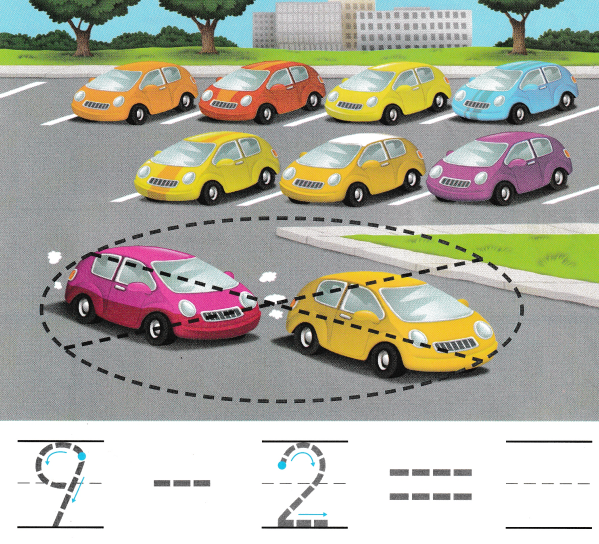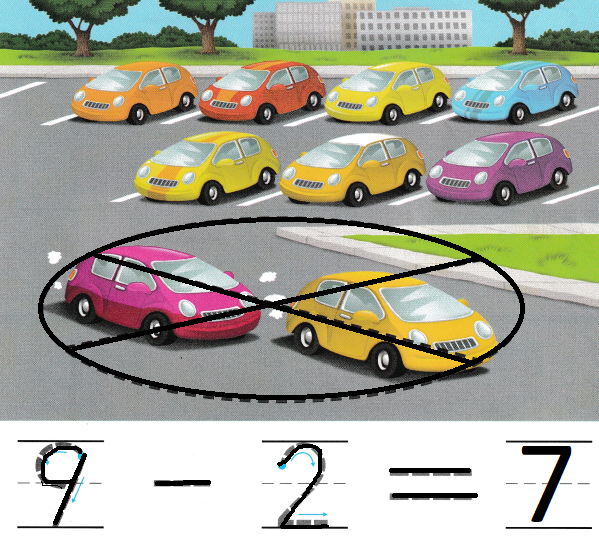Explanation:
There are nine cars.
Two cars drive away.
9 – 2 = 7
so, 7 cars are left
The difference of 9 and 2 is 7

Directions
There are nine cars. Two cars drive away. Trace the circle and X around those cars. How many cars are left? Trace and write to complete the subtraction sentence.

Share and Show

Question 1.Explanation:
There are 8 trucks
3 drove away
8 – 3 = 5
so, 5 trucks are left.

Question 2.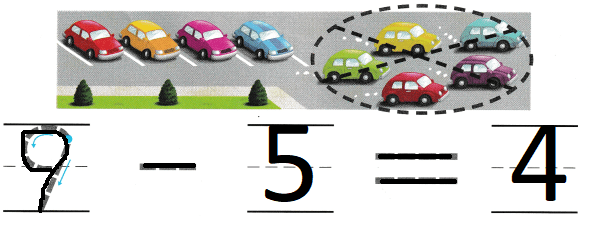Explanation:
There are 9 cars
5 cars drove away
9 – 5 = 4
so, 4 cars are at the parking
The difference of 9 and 5 is 4.

Question 3.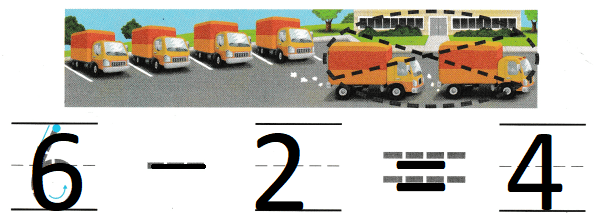Explanation:
There are 6 trucks
out of it 2 trucks driven away
6 – 2 = 4
so, 6 – 2 = 4
so, 4 trucks are left at parking.

Directions 1. Listen to the subtraction word problem. How many ducks swim away? Trace the circle and X. How many ducks are left? Trace and write to complete the subtraction sentence. 2-3. Listen to the subtraction word problem. Trace and write to complete the subtraction sentence.

Question 4.Explanation:
There are 5 fire engines
3 fire engines went to put off the fire
5 – 3 = 2
so, 2 fire engines are left at fire station

Question 5.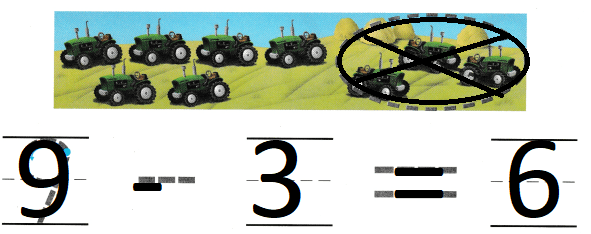Explanation:
There are 9 jeep
3 drive away
9 – 3 = 6
so, jeep are left.

Question 6.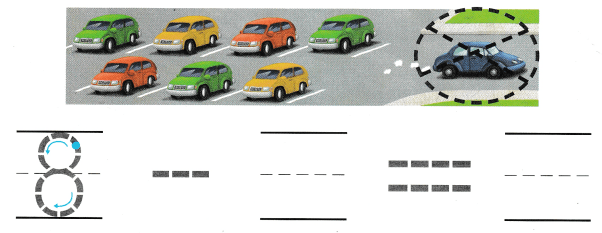Explanation:
there are 8 cars
1 car drive away
8 – 1 = 7
so, 7 cars are left.

Directions
4-6. Listen to the subtraction word problem. Trace and write to complete the subtraction sentence.

Problem Solving

Question 7.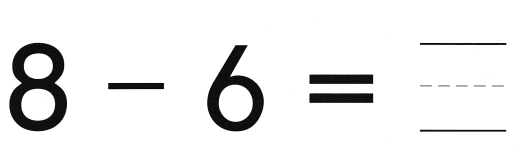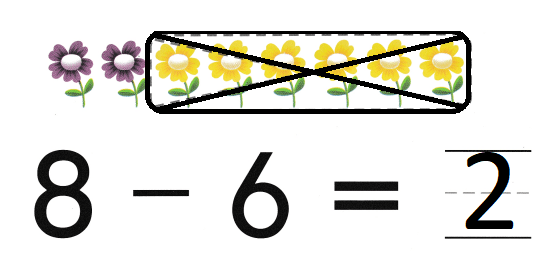Explanation:
There are 8 flowers
6 flowers are yellow
8 – 6 = 2
so, 2 flowers are purple.

Question 8.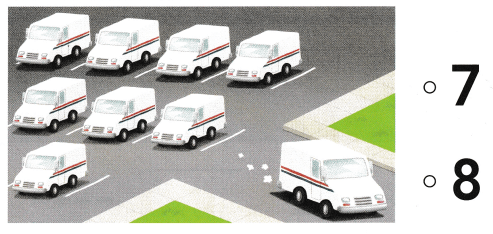Explanation:
There are nine mail trucks.
One mail truck drives away,
9 – 1 = 8
so, 8 trucks are left.

Directions
7. Complete the subtraction sentence. Draw to show what you know about this subtraction sentence. Tell a friend about your drawing. 8. Choose the correct answer. There are nine mail trucks. One mail truck drives away, What is 9 – 1?

### Texas Go Math Kindergarten Lesson 14.2 Homework and Practice

Question 1.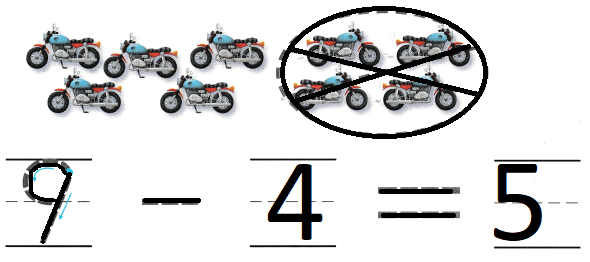Explanation:
There are nine motorcycles.
Four motorcycles drive away
9 – 4 = 5
so, 5 bikes are left.

Question 2.Explanation:
There are eight bicycles.
Four bicycles ride away.
8 – 4 = 4
4 bicycles are left

Directions
Trace and write to complete the subtraction sentence. 1. There are nine motorcycles. Four motorcycles drive away. Trace the circle and X. How many motorcycles are left? 2. There are eight bicycles. Four bicycles ride away. How many bicycles are left?

Texas Test Prep

Lesson Check

Question 3.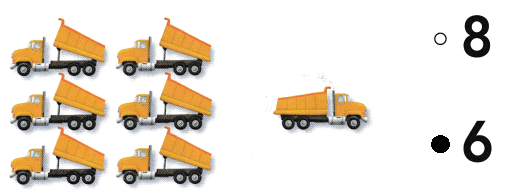Explanation:
There are seven dump trucks.
One dump truck drives away.
7 – 1 = 6
so, 6 dump trunks left.

Question 4.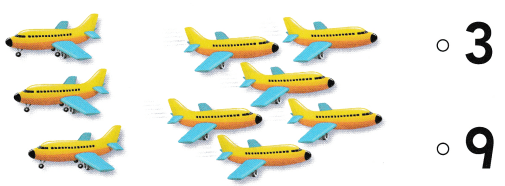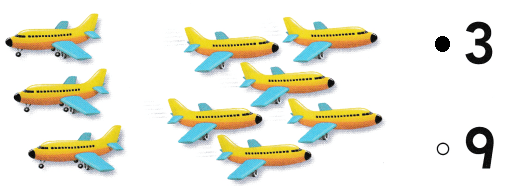Explanation:
There are nine airplanes.
Six airplanes fly away.
9 – 6 = 3
so, 3 planes are left.

Question 5.